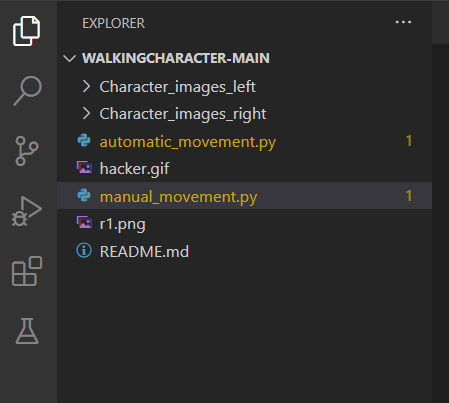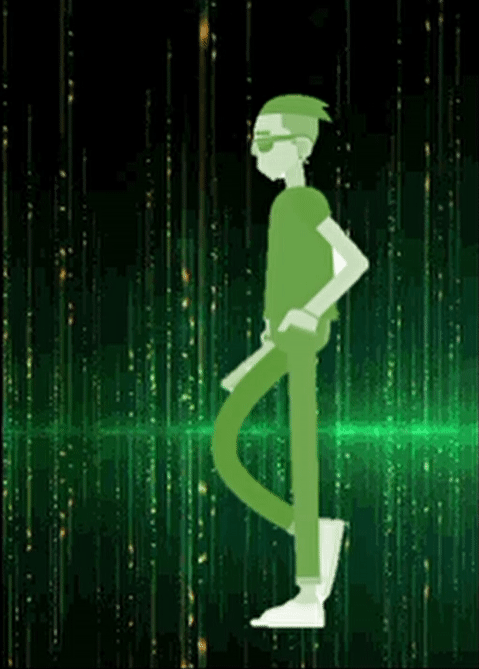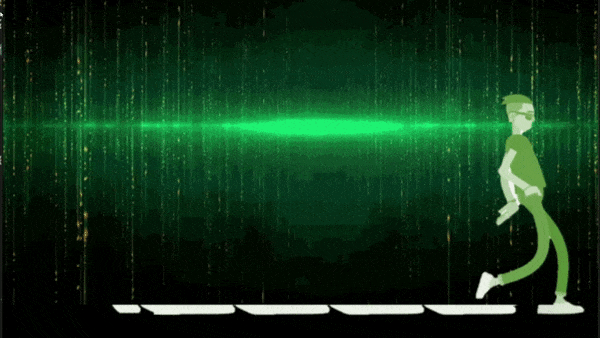# How to create walking character using multiple images from sprite sheet using Pygame?

• Difficulty Level : Medium
• Last Updated : 22 Jun, 2022

In this article, we will cover how to create a walking character using multiple images from a sprite sheet using Pygame

Any video or films that are made are actually the frames of pictures or images. The more the frame of images one has more will be the smoothness of that film or video. In sprite, we can develop any video or game with the use of images. Sprite in Pygame is the feature that provides us to make exciting, creative videos or games with the use of images. Many of us are fond of making our photos like a character in a game. One can also use their images as a character in the game. Here, we will cover how to make a character and we will animate it using time.Clock(). After that, we will implement that code to make it move manually using right-left keys.

Package Requirement

`pip install pygame`

File Structure

To make your code run kindly store your files as in the below-declared file structure.Store the file in the same way as described above

### Example 1: Move character automatically

To move the character in the sprite window without pressing any keyboard keys means the movement of the character automatically.

• Import the below-required libraries.
• Give the dimension of your sprite window and import the collection of your images from your directory.
• Declare a list that can hold each image in one list.
• Provide the movement, velocity, Clock speed, and FPS to your character and you are ready to go with your character.

## Python3

 `import` `pygame as py``import` `os`` ` `# The width and height of our screen``win ``=` `py.display.set_mode((``200``, ``280``))``py.display.set_caption(``"Sprite pygame movement"``)`` ` `# Frames per second``FPS ``=` `30`` ` `# The main class for our sprite window``class` `Sprite_Window(py.sprite.Sprite):``    ``def` `__init__(``self``):``        ``super``(Sprite_Window, ``self``).__init__()`` ` `        ``# Name of the folder in which all the ``        ``# images are stored``        ``folderPath ``=` `"Character_images_left"`` ` `        ``# Storing the folder location in a list``        ``myList ``=` `os.listdir(folderPath)``        ``self``.images ``=` `[]``        ``for` `imPath ``in` `myList:`` ` `            ``# Appending all the images in the array``            ``self``.images.append(py.image.load(f``'{folderPath}/{imPath}'``))`` ` `        ``self``.index ``=` `0``        ``self``.image ``=` `self``.images[``self``.index]``        ``self``.rect ``=` `py.Rect(``5``, ``5``, ``150``, ``198``)`` ` `    ``def` `update(``self``):`` ` `        ``# Increase the value of the index by 1``        ``# so that we can change the sprite images``        ``self``.index ``+``=` `1`` ` `        ``if` `self``.index >``=` `len``(``self``.images):``            ``self``.index ``=` `0``        ``self``.image ``=` `self``.images[``self``.index]`` ` ` ` `py.init()``my_sprite ``=` `Sprite_Window()``my_group ``=` `py.sprite.Group(my_sprite)``clock ``=` `py.time.Clock()`` ` `# q is here for a boolean value to cancel ``# the running window``q ``=` `1``while` `q:``    ``for` `event ``in` `py.event.get():``        ``if` `event.``type` `=``=` `py.QUIT:``            ``q ``=` `0``    ``my_group.update()`` ` `    ``# Background image``    ``bg ``=` `py.image.load(``'hacker.gif'``)`` ` `    ``win.blit(bg, (``1``, ``1``))``    ``my_group.draw(win)``    ``py.display.update()``    ``clock.tick(``13``)`

Output:Output for the automatic walking of a character in sprite

### Example 2: Move the character manually with the right and left keys.

To move the character in the sprite window by pressing the “left-arrow” and “right-arrow” keyboard keys means the movement of the character is manually handled by the user.

• Press the left key to move the character’s left side.
• Press the right key to move the character’s right side.
• You can’t move out of the screen as we have declared the moving direction for the character to not move beyond the displayed screen.

## Python3

 `import` `pygame as py``import` `os``py.init()`` ` `# THE WIDTH AND HEIGHT OF OUR SCREEN``win ``=` `py.display.set_mode((``600``, ``480``))`` ` `py.display.set_caption(``"Sprite pygame direction movement"``)`` ` `# DECLARING THE LOCATION OF THE FOLDER ``# IN WHICH ALL THE LEFT ``# AND RIGHT IMAGES FOR THE MOVEMENT``folderPath_left ``=` `"Character_images_left"``folderPath_right ``=` `"Character_images_right"`` ` `# STORE THE FOLDER LOCATION IN ONE LIST``myList_right ``=` `os.listdir(folderPath_right)``myList_left ``=` `os.listdir(folderPath_left)`` ` `# PICTURE FOR RIGHT MOVEMENT``movement_right ``=` `[]``for` `imPath ``in` `myList_right:``     ` `    ``# APPENDING THE IMAGES WITH THE ``    ``# LOCATION IN A LIST``    ``movement_right.append(py.image.load(f``'{folderPath_right}/{imPath}'``))`` ` `# PICTURE FOR LEFT MOVEMENT``movement_left ``=` `[]``for` `imPath ``in` `myList_left:`` ` `    ``# APPENDING THE IMAGES WITH THE``    ``# LOCATION IN A LIST``    ``movement_left.append(py.image.load(f``'{folderPath_left}/{imPath}'``))`` ` `# SETTING BACKGROUND IMAGE``bg ``=` `py.image.load(``'hacker.gif'``)`` ` `# SETTING CHARACTER IMAGE FOR THE``# STANDING POSITION``standing_position ``=` `py.image.load(``'r1.png'``)`` ` `x ``=` `20``y ``=` `100``width ``=` `40``height ``=` `100``velocity ``=` `5`` ` `clock ``=` `py.time.Clock()`` ` `left ``=` `False``right ``=` `False``movement_count ``=` `0`` ` `# Declaring the sprite window``def` `Sprite_Window():``   ` `    ``# To store the movement count``    ``global` `movement_count`` ` `    ``win.blit(bg, (``1``, ``1``))``    ``if` `movement_count ``+` `1` `>``=` `20``:``        ``movement_count ``=` `0`` ` `    ``# To move the character right side of ``    ``# the sprite window``    ``if` `right:``        ``win.blit(movement_right[movement_count``/``/``4``], (x, y))``        ``movement_count ``=` `movement_count ``+` `1`` ` `    ``# To move the character left side of ``    ``# the sprite window``    ``elif` `left:``        ``win.blit(movement_left[movement_count``/``/``4``], (x, y))``        ``movement_count ``=` `movement_count ``+` `1`` ` `    ``# If user will not press any keys then``    ``# the image in the sprite``    ``# window will be in the standing position``    ``else``:``        ``win.blit(standing_position, (x, y))``        ``movement_count ``=` `0`` ` `    ``# To update the display screen``    ``py.display.update()`` ` ` ` `# This will be used to quit the window``run ``=` `1``while` `run:``   ` `    ``# This will manage the frame rate and provide ``    ``# more smoothness to the movement``    ``clock.tick(``33``)``    ``for` `event ``in` `py.event.get():``        ``if` `event.``type` `=``=` `py.QUIT:``            ``run ``=` `0``    ``keys ``=` `py.key.get_pressed()`` ` `    ``# To move to the end of the screen``    ``if` `keys[py.K_RIGHT] ``and` `x < ``480` `-` `velocity ``-` `width:``       ` `        ``# For moving in the right direction``        ``x ``+``=` `velocity``        ``right ``=` `True``        ``left ``=` `False``         ` `    ``# To move to the end of the screen``    ``elif` `keys[py.K_LEFT] ``and` `x > velocity ``-` `width:``       ` `        ``# For moving in the left direction``        ``x ``-``=` `velocity``        ``right ``=` `False``        ``left ``=` `True``    ``else``:``        ``left ``=` `False``        ``right ``=` `False``        ``movement_count ``=` `0`` ` `    ``Sprite_Window()``py.quit()`

Output:Output for the manual movement of the character by the left and right key in sprite

My Personal Notes arrow_drop_up